## 7.1.2 Work done in a plastic hinge

Let us consider the internal energy in the plastic hinge from Fig. 7.5(a):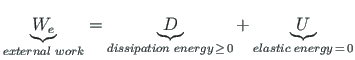(7.11)

where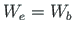is the work done by external forces (see Appendix B.1).

The work done by external forces at Sign Convention 1 (see Fig. 7.5(a))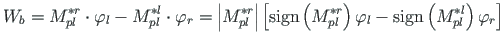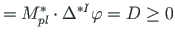(7.12)

The work done by external forces at Sign Convention 2 (see Fig. 7.5(b))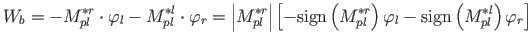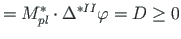(7.13)

The incremental rotation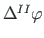of the plastic hinge is given by the difference between rotations of the member ends at joint. It is necessary to check the compatibility of the rotations related to the hinge with the sign of the plastic moment of Eq. (7.13).

Considering that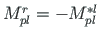and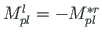, the work of boundary forces at the member ends at joint is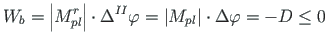(7.14)

where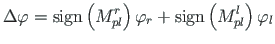(7.15)[Sign Convention 1][Sign Convention 2]

The boundary forces work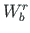at the member end and the boundary forces work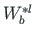at the plastic hinge boundary, at a similar angle of rotation,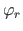, have different signs (see Fig. 7.5(b)):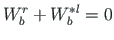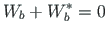(7.16)

The sum of the amounts of work done by internal and external forces is zero (see Appendix B.1 and Eq. (B.8)), or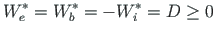(7.17)

and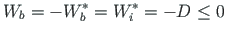(7.18)

The sum of the amounts of work done by boundary forces of members at the plastic hinge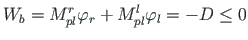(7.19)

andres
2014-09-09